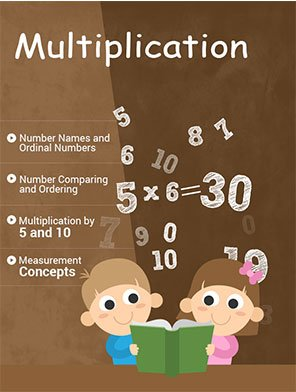\$7.00

## MATH MULTIPLICATION WORKSHEETS

In our math multiplication worksheets, students are introduced to the concepts of multiplication as a repeated addition. The separate multiplication table of 2 to 10 in different exercises will describe how multiplication is related with the addition. Same as addition and subtraction, students begin multiplication exercise, multiplying 2 to all numbers up to 10. Similarly, they will continue for numbers 5, 10, 3, 4, and onward. Each exercise in multiplication will increase in variety and complexity to make students efficient in multiplication concepts. Multiplying 2 or 3 digit numbers by 1 or 2 digit numbers and multiplication by tens, by hundreds, and by thousands are the advanced problems in 15 units long multiplication section.

Worksheet # Topics/Curriculum # of Pages 2pg Sample Add to cart
Unit-1 Multiplication by 2, by 5, and by 10 8 pages\$1.00

Unit-2 Multiplication by 3, by 4, and by 6 8 pages\$1.00

Unit-3 Multiplication by 7, by 8, and by 9 8 pages\$1.00

Unit-4 Multiplication up to 10 8 pages\$1.00

Unit-5 Multiplying 2 Digit Numbers by 2, by 3, and by 4 8 pages\$1.00

Unit-6 Multiplying 2 Digit Numbers by 2 through 7 8 pages\$1.00

Unit-7 Multiplying 2 Digit Numbers by 2 through 9 8 pages\$1.00

Unit-8 Multiplying 2 Digit Numbers Ending in 0 or 5 8 pages\$1.00

Unit-9 Multiplying 2 Digit Numbers by 1 Digit Numbers 8 pages\$1.00

Unit-10 Multiplying 3 or More Digit Numbers by 1 Digit Numbers 8 pages\$1.00

Unit-11 Multiplying 2-Digit Numbers by 2-Digit Numbers Part I 8 pages\$1.00

Unit-12 Multiplying 2-Digit Numbers by 2-Digit Numbers Part II 8 pages\$1.00

Unit-13 Multiplying 2 or 3 Digit Numbers by 2-Digit Numbers 8 pages\$1.00

Unit-14 Multiplying 2 or 3 Digit Numbers by 1 or 2 Digit Numbers 8 pages\$1.00

Unit-15 More Problems on Multiplication 8 pages\$1.00